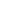Functions

 Data Component Functions Return components of 3D data structures

 RtFloat N3D_XComp(RtFloat *theVector) RtFloat N3D_YComp(RtFloat *theVector) RtFloat N3D_ZComp(RtFloat *theVector) RtFloat N3D_WComp(RtFloat *theVector)

 Data Conversion Functions Convert between RtPoints and RtBounds

 void N3D_ConvertBoundToPoints(RtBound theBound, RtPoint * thePoints) void N3D_ConvertPointsToBound(RtPoint * thePoints, RtBound theBound)

 Data Copying Functions Efficiently copy 3D data types

 void N3D_CopyBound(RtBound sourceBounds, RtBound destBounds) void N3D_CopyMatrix(RtMatrix sourceMatrix, RtMatrix destMatrix) void N3D_CopyPoint(RtPoint sourcePoint, RtPoint destPoint)

 Intersection Testing Function Test for intersection between line and plane

 void N3DIntersectLinePlane(RtPoint *endPoints, RtPoint planeNormal, RtPoint planePoint, RtPoint *intersection)

 Matrix Manipulation Functions Efficient matrix multiplication

 void N3DMultiplyMatrix(RtMatrix preTransform, RtMatrix postTransform, RtMatrix resultTransform) float N3DInvertMatrix(RtMatrix theTransform, RtMatrix theInverse)

 Transformation Functions Transform between coordinate systems

 void N3DMult3DPoint(RtPoint thePoint, RtMatrix theTransform, RtPoint newPoint) void N3DMult3DPoints(RtPoint *thePoints, int pointCount,RtMatrix theTransform, RtPoint *newPoints)